# SSAT Elementary Level Math : Rectangles

## Example Questions

← Previous 1 3 4 5 6 7 8 9 52 53

### Example Question #1 : How To Find The Area Of A Rectangle

Aaron and his family just moved into a new house. He wants to figure out the size of his new room. It is a rectangle. One side is 12 feet and the other side is 10 feet. What is the area of his new room?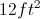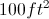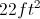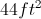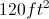Explanation:

To find the area of a rectangle, multiply the length by the width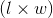.

The question tells us that the length is 12 feet and the width is 10 feet.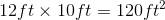### Example Question #2 : How To Find The Area Of A Rectangle

If Tim draws a rectangle with a long side of 8 mm and a short side of 4 mm, what is the area?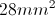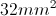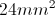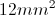Explanation:

To find the area of a rectangle, multiply the length (8mm) by the width (4mm).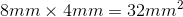Therefore, the area is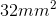.

### Example Question #1 : How To Find The Area Of A Rectangle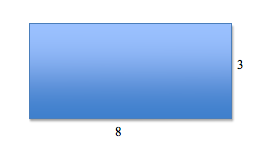What is the area of the above rectangle?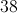Explanation:

To find the area of the rectangle, multiply the longer side (8) and shorter side (3) together.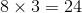### Example Question #4 : How To Find The Area Of A Rectangle

John needs a tablecloth that covers his entire dining room table. The tabletop measures 4 feet by 7 feet. Which of the following tablecloths should John buy?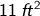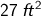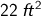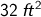Explanation:

The tablecloth measurements are areas, shown by the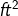units in the answer choices. Since the tabletop is 4 ft by 7 ft, John needs a tablecloth with an area of AT LEAST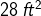.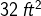is the only answer choice that meets that requirement.

### Example Question #1 : How To Find The Area Of A Rectangle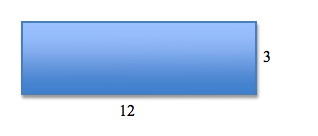What is the area of the above rectangle?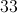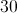Explanation:

To find the area of the rectangle, multiply the longer side (12) and the shorter side (3) together.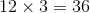### Example Question #1 : Rectangles

A rectangle has a perimeter of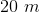. One of its sides has a length of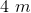.

What is the area of the rectangle?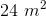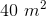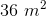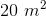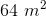Explanation:

The perimeter of a figure is the sum of the lengths of all its sides. Every rectangle has two sets of two equal-length sides. One set of sides iseach, or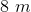when added together.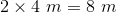We subtractfrom the totalperimeter to find the lengths of the remaining sides.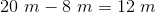Since there are two remaining sides, the length of each of the remaining sides must be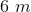.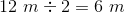The area of a rectangle is given by multiplying the length of its longer side by the length of its shorter side.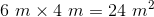### Example Question #7 : How To Find The Area Of A Rectangle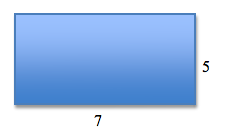What is the area of the above rectangle?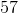Explanation:

To find the area of the rectangle, multiply the longer side (7) and the shorter side (5) together.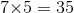### Example Question #8 : How To Find The Area Of A Rectangle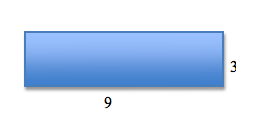What is the area of the rectangle?Explanation:

To find the area of a rectangle, multiply the width by the length.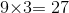### Example Question #9 : How To Find The Area Of A Rectangle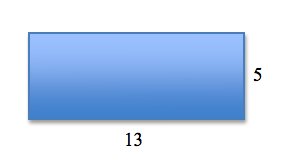What is the area of the rectangle?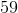Explanation:

To find the area of a rectangle, multiply the length of the longer side by the shorter side.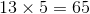### Example Question #1 : How To Find The Area Of A Rectangle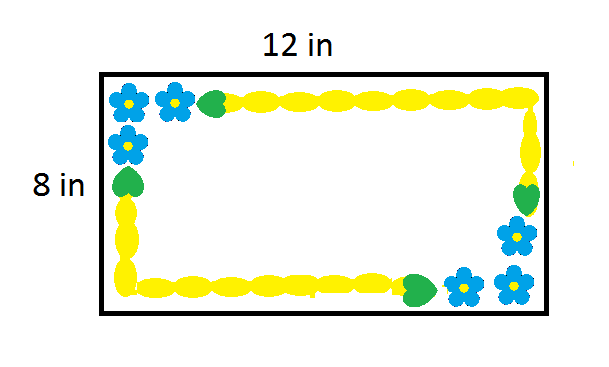A rectangular birthday cake (shown in the diagram) measures 8 inches by 12 inches. The cake is then divided into four identical rectangular slices. What is the area of each slice?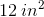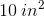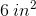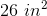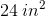Explanation: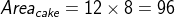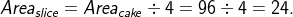← Previous 1 3 4 5 6 7 8 9 52 53

### All SSAT Elementary Level Math Resources